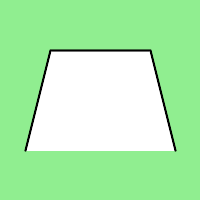Skip to content
Related Articles
Wand polyline() function in Python
• Last Updated : 10 May, 2020

polyline() is another drawing function present in wand.drawing module of Wand. polyline() is similar to polygon() function the only difference is that, it will not close the stroke line b/w first and last point. Similar to polygon() it also takes a list of point tuples as an argument.

Syntax :

```wand.drawing.polyline(points)
```

Parameters :

ParameterInput TypeDescription
pointslistlist of x, y tuples.

Example #1

 `from` `wand.image ``import` `Image``from` `wand.drawing ``import` `Drawing``from` `wand.color ``import` `Color`` ` `with Drawing() as draw:``    ``draw.stroke_width ``=` `2``    ``draw.stroke_color ``=` `Color(``'black'``)``    ``draw.fill_color ``=` `Color(``'white'``)`` ` `    ``# points list for polygon``    ``points ``=` `[(``25``, ``25``), (``175``, ``100``), (``25``, ``175``)]`` ` `    ``# draw polygon using polygon() function``    ``draw.polyline(points)``    ``with Image(width ``=` `200``, height ``=` `200``, background ``=` `Color(``'lightgreen'``)) as image:``        ``draw(image)``        ``image.save(filename ``=` `"polygon.png"``)`

Output:Example #2:

 `from` `wand.image ``import` `Image``from` `wand.drawing ``import` `Drawing``from` `wand.color ``import` `Color`` ` `with Drawing() as draw:``    ``draw.stroke_width ``=` `2``    ``draw.stroke_color ``=` `Color(``'black'``)``    ``draw.fill_color ``=` `Color(``'white'``)`` ` `    ``# points list for polygon``    ``points ``=` `[(``25``, ``150``), (``50``, ``50``), (``150``, ``50``), (``175``, ``150``)]`` ` `    ``# draw polygon using polygon() function``    ``draw.polyline(points)``    ``with Image(width ``=` `200``, height ``=` `200``, background ``=` `Color(``'lightgreen'``)) as image:``        ``draw(image)``        ``image.save(filename ``=` `"polyline2.png"``)`

Output:Attention geek! Strengthen your foundations with the Python Programming Foundation Course and learn the basics.

To begin with, your interview preparations Enhance your Data Structures concepts with the Python DS Course. And to begin with your Machine Learning Journey, join the Machine Learning – Basic Level Course

My Personal Notes arrow_drop_up